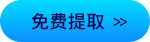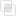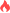python爬虫设置定时任务的三种方法

Python爬虫2021-03-25 14:15:48696浏览 · 0收藏 · 0评论```import os
import time
import sys
from datetime import datetime, timedelta
def One_Plan():
# 设置启动周期
Second_update_time = 24 * 60 * 60

# 当前时间
now_Time = datetime.now()
# 设置 任务启动时间
plan_Time = now_Time.replace(hour=9, minute=0, second=0, microsecond=0)
# 设置差值，-1 day, 21:48:53.246576，类似于这样
# time.sleep()需要传入int，所以下面使用.total_seconds()
# 主要用来计算差值，返回int，具体功能可以自行查阅相关资料
delta = plan_Time - now_Time
first_plan_Time = delta.total_seconds() % Second_update_time
print("距离第一次执行需要睡眠%d秒" % first_plan_Time)
return first_plan_Time

# while Ture代码块，挂起程序，睡眠时间结束后调用函数名进行执行
while True:

s1 = One_Plan()
time.sleep(s1)
# 下面这里是自己定义的函数，想跑代码的可以换成hellow world函数或者注释掉这行测试下
exe_file(D_list)
print("正在执行首次更新程序")```

```from datetime import datetime
from threading import Timer
import time

# 定时任务
def task():
print(datetime.now().strftime("%Y-%m-%d %H:%M:%S"))

def timedTask():
'''
第一个参数: 延迟多长时间执行任务(秒)
第二个参数: 要执行的函数
第三个参数: 调用函数的参数(tuple)
'''
Timer(5, task, ()).start()

while True:
timedTask()
time.sleep(5)```

```import schedule
import time

def hellow():
print('hellow')

def Timer():
schedule.every().day.at("09:00").do(hellow)
schedule.every().day.at("18:00").do(hellow)

while True:
schedule.run_pending()

time.sleep('需要睡眠的周期')

Timer()```

（推荐操作系统：windows7系统、Python 3.9.1，DELL G3电脑。）python中的Where函数怎么用？python中Rank函数怎么用？如何正确并快速安装python库？python爬虫中使用正则match( )方法匹配目标python中xlwings是什么？MongoDB中find()和findOne()有何不同？869

MongoDB中findOne()方法使用详解593

python中if嵌套命令如何理解？1155